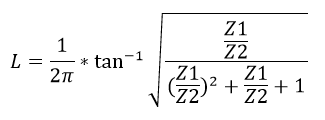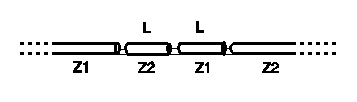# Bramham Twelfth Wave Transformer Calculator

This CalcTown calculator calculates the precise electrical length of the twelfth-wave section.

The Twelfth-Wave Transformer is often a more convenient alternative to the more well-known quarter-wave transformer. It is not a new concept, being first publilshed in 1961, but it is relatively unknown in amateur radio circles.

With the twelfth-wave transformer, two lengths of cable are used in series, each electrically nearly one twelfth-wavelength, but of characteristic impedances equal to the two impedances Z1 and Z2 being matched. The figures below illustrate the difference between the twelfth-wave and a quarter-wave transformer.

The SWR bandwidth of the twelfth-wave transformer is very broad, and is comparable to the quarter-wave transformer.

#### Result

WavelengthsClick here to view image

Where,

Z1 = impedance 1 to be matched

Z2 = impedance 2 to be matched

L = electrical length of the twelfth-wave section